### My HeroHover over the thumbnail for a full-size version.

Author wedgie123 author:wedgie123 bitesized n-art rated 2005-12-24 4 by 467 people. \$My Hero#wedgie123#none#00000000000000000000000000000000000000000000000000000000000000000000000000000000000000000000000000000000000000000000000000000000000000000000000000000000000000000000000000000000000000000000000000000000000000000000000000000000000000000000000000000000000000000000000000000000000000000000000000000000000000000000000000000000000000000000000000000000000000000000000000000000000000000000000000000000000000000000000000000000000000000000000000000000000000000000000000000000000000000000000000000000000000000000000000000000000000000000000000000000000000000000000000000000000000000000000000000000000000000000000000000000000000000000000000000000000000000000000000000000000000000000000000000000000000000000000000000000000000000|0^192,333!0^193,352!0^620,412!0^625,257!0^629,236!0^631,201!0^629,179!0^620,138!0^618,131!0^190,295!0^187,277!0^185,241!0^182,169!12^625,483!12^635,464!12^648,442!12^656,422!12^663,400!12^664,385!12^676,364!12^677,332!12^677,304!12^681,281!12^685,254!12^685,237!12^685,215!12^689,188!12^691,164!12^692,140!12^692,118!12^686,87!12^679,61!12^667,24!12^216,455!12^213,466!12^213,479!12^206,485!12^196,493!12^191,477!12^190,457!12^190,441!12^182,453!12^176,457!12^169,459!12^183,411!12^175,428!12^173,438!12^170,448!12^165,452!12^158,456!12^155,438!12^172,390!12^168,395!12^160,401!12^153,410!12^142,419!12^131,425!12^121,430!12^111,436!12^166,388!12^153,398!12^142,401!12^134,405!12^112,411!12^154,349!12^149,356!12^143,367!12^137,376!12^121,386!12^109,388!12^140,379!12^145,376!12^159,365!12^191,465!12^191,471!12^193,483!12^193,488!12^142,447!12^161,456!12^166,449!12^170,444!12^176,436!12^176,427!12^177,423!12^179,416!12^173,433!12^171,439!12^161,431!12^160,432!12^160,433!12^157,435!12^157,435!12^164,426!12^171,421!12^178,415!12^178,411!12^180,408!12^114,433!12^120,432!12^120,430!12^123,429!12^131,426!12^132,426!12^134,424!12^140,421!12^142,419!12^143,418!12^148,416!12^148,415!12^148,414!12^169,398!12^147,426!12^150,424!12^150,421!12^157,416!12^173,406!12^176,405!12^196,379!12^195,380!12^194,381!12^188,386!12^186,387!12^182,391!12^176,398!12^176,401!12^178,401!12^190,390!12^193,389!12^195,387!12^195,386!12^195,387!12^195,389!12^195,390!12^195,391!12^195,393!12^198,394!12^198,397!12^198,397!12^196,399!12^191,400!12^190,400!12^186,404!12^184,404!12^182,403!12^178,405!12^195,403!12^194,406!12^193,407!12^192,409!12^192,412!12^192,420!12^192,420!12^192,423!12^192,429!12^192,432!12^192,432!12^192,435!12^192,438!12^192,439!12^192,442!12^192,444!12^192,446!12^192,450!12^192,450!12^203,417!12^203,421!12^201,422!12^197,426!12^194,432!12^193,436!12^191,439!12^189,444!12^189,444!12^187,446!12^187,447!12^187,447!12^180,454!12^179,455!12^192,407!12^190,412!12^189,417!12^187,423!12^187,426!12^186,429!12^185,430!12^184,433!12^183,434!12^181,436!12^180,437!12^175,442!12^175,442!12^174,445!12^171,447!12^180,441!12^200,418!12^200,416!12^199,414!12^199,410!12^199,410!12^198,406!12^204,450!12^203,451!12^202,452!12^200,455!12^198,459!12^195,466!12^194,469!12^193,472!12^211,454!12^210,458!12^208,463!12^207,467!12^205,476!12^204,479!12^203,484!12^203,486!12^203,490!12^203,492!12^203,496!12^203,499!12^196,486!12^196,481!12^199,475!12^199,473!12^202,461!12^202,471!12^201,477!12^201,478!12^203,460!12^205,453!12^205,453!12^205,467!12^202,430!12^202,431!12^202,437!12^202,450!12^201,429!12^200,427!12^157,428!12^156,428!12^154,428!12^150,428!12^145,428!12^135,430!12^133,430!12^165,445!12^147,449!12^173,372!12^172,372!12^170,376!12^169,376!12^168,376!12^165,380!12^165,380!12^162,382!12^160,383!12^157,386!12^156,387!12^154,389!12^150,390!12^150,390!12^146,395!12^144,395!12^141,396!12^139,398!12^124,403!12^119,403!12^115,403!12^112,403!12^152,389!12^149,393!12^148,393!12^144,397!12^140,401!12^138,402!12^133,406!12^124,412!12^119,414!12^114,417!12^111,415!12^104,404!12^85,401!12^99,419!12^91,417!12^83,416!12^104,412!12^96,411!12^105,420!12^107,419!12^165,390!12^163,391!12^161,394!12^157,398!12^153,400!12^149,402!12^147,404!12^144,409!12^133,414!12^96,404!12^126,416!12^154,352!12^148,362!12^147,365!12^128,380!12^119,384!12^155,357!12^155,356!12^155,363!12^145,368!12^156,365!12^141,375!12^138,376!12^116,385!12^160,368!12^160,371!12^156,371!12^146,372!12^163,376!12^161,377!12^159,379!12^154,381!12^152,384!12^150,385!12^146,389!12^144,392!12^139,397!12^151,374!12^190,378!12^188,380!12^186,380!12^183,380!12^180,377!12^178,376!12^177,374!12^174,374!12^174,374!12^173,380!12^167,384!12^165,384!12^168,383!12^174,383!12^176,384!12^177,386!12^117,418!12^116,418!12^129,404!12^108,407!12^103,405!12^144,288!12^144,292!12^145,295!12^145,296!12^149,299!12^150,302!12^150,303!12^183,340!12^181,335!12^172,325!12^170,321!12^165,315!12^157,308!12^152,295!12^177,332!12^189,305!12^189,302!12^184,294!12^181,285!12^180,279!12^176,270!12^172,266!12^138,288!12^132,289!12^129,289!12^122,289!12^100,318!12^100,318!12^103,311!12^105,306!12^107,302!12^108,299!12^115,294!12^119,289!12^120,289!12^111,294!12^118,140!12^114,180!12^96,246!12^81,259!12^118,87!12^118,124!12^118,127!12^118,133!12^118,140!12^118,150!12^118,154!12^118,161!12^116,178!12^110,199!12^108,210!12^107,218!12^105,222!12^104,226!12^103,229!12^101,230!12^99,238!12^99,240!12^97,244!12^96,247!12^95,250!12^94,251!12^91,254!12^87,257!12^84,261!12^119,241!12^114,244!12^112,246!12^103,249!12^99,250!12^94,253!12^86,259!12^86,259!12^86,260!12^109,249!12^118,244!12^116,249!12^112,253!12^109,258!12^107,261!12^102,266!12^97,269!12^93,272!12^97,284!12^99,284!12^104,281!12^106,277!12^108,271!12^112,266!12^113,261!12^115,256!12^115,252!12^91,289!12^94,288!12^95,287!12^100,285!12^104,283!12^108,282!12^114,279!12^119,276!12^120,275!12^119,278!12^116,284!12^114,287!12^111,291!12^109,294!12^111,297!12^112,272!12^114,270!12^117,265!12^118,258!12^119,251!12^104,241!12^107,234!12^108,231!12^104,235!12^109,235!12^72,265!12^58,267!12^80,264!12^81,291!12^66,294!12^95,325!12^89,382!12^74,394!12^145,306!12^149,310!12^152,311!12^156,319!12^166,327!12^138,298!12^134,295!12^126,297!12^110,311!12^118,301!12^216,84!12^216,108!12^228,168!12^234,181!12^232,176!12^224,156!12^219,139!12^215,120!12^215,96!12^221,148!12^215,129!12^206,165!12^195,160!12^185,151!12^183,167!12^180,166!12^216,163!12^211,156!12^206,148!12^201,138!12^192,119!12^195,127!12^184,84!12^283,96!12^271,96!12^249,86!12^242,81!12^237,79!12^257,89!12^329,100!12^301,96!12^277,88!12^290,91!12^316,100!12^322,100!12^307,98!12^295,95!12^278,89!12^273,86!12^261,82!12^259,80!12^251,76!12^267,82!12^283,87!12^406,109!12^391,101!12^375,89!12^371,83!12^356,62!12^348,45!12^341,29!12^368,82!12^360,83!12^351,83!12^339,82!12^325,79!12^328,86!12^319,82!12^314,77!12^324,100!12^320,99!12^314,96!12^311,92!12^305,90!12^303,88!12^291,77!12^286,74!12^298,84!12^305,82!12^304,79!12^294,71!12^241,95!12^225,82!12^187,308!12^179,300!12^173,297!12^169,294!12^162,288!12^185,325!12^184,325!12^180,324!12^176,321!12^172,316!12^168,309!12^166,306!12^165,302!12^164,299!12^162,294!12^157,296!12^157,299!12^160,308!12^170,318!12^185,330!12^185,333!12^185,334!12^185,336!12^192,337!12^192,340!12^192,348!12^192,350!12^192,351!12^193,365!12^195,376!12^195,378!12^196,385!12^199,391!12^189,303!12^189,296!12^189,291!12^188,284!12^185,278!12^185,273!12^185,265!12^185,262!12^185,254!12^185,243!12^182,239!12^180,233!12^179,229!12^178,223!12^178,212!12^178,204!12^178,194!12^224,90!12^224,98!12^225,113!12^234,90!12^334,69!12^340,69!12^349,67!12^351,66!12^352,66!12^354,65!12^336,75!12^336,75!12^341,75!12^341,75!12^349,75!12^351,78!12^355,78!12^359,78!12^361,78!12^364,78!12^343,76!12^330,59!12^306,44!12^298,26!12^298,32!12^301,38!12^309,48!12^313,52!12^317,56!12^321,56!12^327,57!12^334,57!12^342,41!12^334,31!12^331,29!12^328,28!12^326,26!12^307,30!12^323,52!12^310,40!12^344,82!12^330,82!12^320,82!12^335,81!12^312,80!12^396,104!12^384,97!12^378,92!12^361,72!12^358,68!12^354,57!12^353,51!12^347,37!12^347,33!12^346,31!12^344,25!12^350,48!12^349,46!12^376,81!12^388,80!12^397,77!12^414,70!12^423,75!12^411,75!12^403,73!12^399,73!12^393,73!12^387,71!12^384,70!12^381,70!12^376,69!12^370,68!12^367,66!12^391,39!12^399,51!12^412,63!12^421,65!12^380,36!12^376,50!12^369,45!12^369,34!12^390,56!12^353,36!12^353,40!12^356,40!12^357,41!12^357,45!12^357,48!12^357,53!12^359,58!12^353,48!12^353,40!12^351,36!12^358,38!12^358,38!12^359,33!12^346,27!12^350,27!12^420,66!12^420,66!12^416,65!12^414,63!12^407,59!12^405,57!12^404,54!12^403,53!12^401,51!12^397,46!12^395,43!12^393,40!12^389,37!12^388,33!12^387,32!12^387,31!12^421,62!12^419,61!12^417,60!12^415,57!12^414,56!12^411,54!12^410,53!12^408,50!12^406,46!12^404,44!12^403,43!12^400,41!12^397,33!12^395,31!12^402,46!12^402,50!12^402,53!12^401,46!12^397,42!12^393,38!12^389,34!12^388,31!12^388,29!12^391,30!12^393,31!12^396,32!12^406,57!12^410,57!12^413,57!12^146,325!12^144,316!12^378,77!12^376,77!12^375,77!12^372,74!12^369,72!12^365,70!12^363,65!12^362,62!12^384,93!12^379,88!12^376,85!12^387,97!12^384,66!12^381,66!12^379,65!12^377,62!12^374,59!12^368,53!12^366,50!12^365,48!12^363,42!12^363,38!12^363,58!12^371,66!12^372,66!12^373,65!12^370,60!12^363,55!12^362,51!12^360,46!12^386,35!12^383,35!12^378,32!12^371,31!12^366,30!12^362,30!12^356,28!12^354,26!12^357,24!12^360,24!12^369,24!12^375,24!12^378,24!12^387,24!12^393,24!12^393,26!12^378,26!12^369,26!12^363,26!12^379,27!12^391,27!12^368,29!12^368,28!12^383,28!12^383,28!12^373,28!12^376,41!12^397,59!12^383,53!12^372,37!12^397,57!12^387,59!12^381,63!12^386,44!12^398,65!12^391,65!12^410,65!12^482,99!12^467,93!12^456,87!12^446,78!12^434,74!12^476,96!12^474,96!12^466,95!12^463,92!12^459,91!12^455,87!12^451,83!12^449,81!12^447,79!12^446,76!12^443,73!12^436,73!12^433,73!12^429,73!12^425,73!12^420,73!12^416,73!12^534,97!12^517,99!12^504,100!12^497,102!12^526,98!12^508,98!12^492,98!12^492,102!12^486,102!12^511,100!12^504,101!12^524,98!12^225,162!12^221,153!12^217,146!12^217,140!12^215,135!12^214,128!12^214,124!12^213,113!12^214,111!12^214,107!12^214,98!12^214,93!12^214,83!12^214,82!12^214,104!12^212,117!12^192,158!12^184,151!12^184,150!12^201,120!12^198,119!12^196,116!12^193,114!12^189,112!12^186,112!12^186,117!12^186,119!12^186,122!12^185,125!12^185,128!12^185,134!12^185,142!12^186,149!12^186,151!12^191,153!12^189,149!12^185,143!12^178,159!12^178,166!12^193,113!12^200,116!12^204,117!12^208,117!12^209,113!12^208,108!12^206,101!12^206,96!12^206,93!12^206,91!12^206,89!12^207,122!12^212,126!12^212,129!12^202,108!12^199,109!12^197,108!12^191,107!12^190,100!12^195,94!12^199,93!12^202,93!12^202,92!12^198,93!12^198,103!12^200,105!12^201,105!12^199,114!12^195,113!12^195,102!12^201,101!12^204,101!12^206,100!12^236,89!12^235,87!12^233,84!12^231,82!12^227,75!12^225,71!12^224,91!12^224,87!12^224,83!12^221,81!12^217,79!12^215,69!12^213,90!12^213,100!12^212,104!12^210,92!12^209,82!12^216,86!12^217,85!12^202,137!12^198,132!12^196,130!12^193,126!12^202,124!12^208,124!12^189,127!12^208,134!12^200,129!12^210,141!12^304,92!12^301,90!12^295,87!12^287,85!12^282,83!12^281,83!12^279,81!12^273,79!12^268,78!12^262,76!12^260,75!12^257,75!12^288,92!12^285,91!12^282,88!12^279,84!12^274,82!12^269,78!12^299,90!12^297,90!12^293,89!12^292,86!12^290,83!12^287,81!12^285,78!12^275,75!12^268,71!12^265,71!12^264,71!12^262,73!12^251,73!12^244,73!12^241,73!12^238,73!12^233,73!12^232,73!12^231,73!12^219,75!12^216,75!12^213,75!12^211,75!12^243,74!12^236,74!12^227,74!12^226,70!12^228,67!12^230,66!12^234,66!12^242,65!12^252,65!12^257,65!12^258,65!12^262,66!12^269,69!12^264,75!12^259,69!12^257,68!12^254,68!12^249,68!12^245,68!12^242,68!12^233,68!12^229,68!12^319,171!12^313,173!12^305,175!12^299,177!12^294,176!12^280,159!12^284,158!12^285,164!12^285,167!12^287,172!12^286,176!12^290,177!12^324,170!12^329,167!12^334,162!12^333,157!12^330,153!12^325,152!12^316,149!12^309,149!12^298,150!12^288,152!12^281,153!12^272,155!12^246,178!12^243,184!12^252,183!12^261,180!12^266,178!12^302,150!12^292,150!12^274,154!12^248,175!12^250,184!12^257,179!12^251,179!12^252,175!12^252,170!12^257,167!12^260,166!12^265,163!12^267,161!12^259,166!12^259,168!12^259,169!12^260,171!12^260,177!12^271,160!12^276,157!12^246,183!12^264,171!12^320,149!12^288,199!12^248,172!12^352,152!12^340,149!12^328,149!12^335,149!12^347,152!12^360,174!12^354,166!12^350,158!12^355,169!12^350,162!12^351,156!12^348,183!12^353,177!12^343,195!12^299,196!12^315,192!12^329,184!12^339,174!12^293,183!12^308,182!12^325,177!12^316,179!12^300,180!12^283,184!12^274,182!12^270,182!12^338,155!12^344,156!12^344,161!12^340,164!12^340,159!12^335,155!12^352,174!12^352,172!12^349,167!12^343,165!12^340,167!12^335,167!12^333,167!12^348,175!12^344,174!12^338,174!12^330,174!12^342,168!12^342,184!12^334,179!12^334,191!12^325,190!12^319,183!12^325,204!12^305,204!12^305,185!12^304,171!12^299,174!12^296,174!12^293,172!12^293,166!12^291,165!12^290,162!12^289,159!12^289,159!12^295,159!12^298,155!12^290,156!12^309,154!12^310,154!12^315,154!12^317,154!12^318,154!12^318,161!12^316,166!12^311,166!12^308,166!12^304,166!12^300,166!12^297,164!12^298,159!12^301,156!12^306,157!12^306,161!12^306,162!12^292,154!12^311,158!12^314,158!12^315,158!12^297,169!12^303,162!12^312,162!12^291,171!12^309,173!12^362,140!12^346,140!12^330,136!12^310,134!12^286,133!12^269,137!12^250,146!12^238,161!12^365,140!12^378,133!12^381,123!12^369,117!12^348,117!12^326,113!12^310,109!12^291,107!12^274,111!12^257,120!12^245,128!12^232,140!12^371,136!12^353,138!12^320,134!12^317,134!12^299,132!12^292,132!12^274,134!12^278,134!12^257,141!12^242,154!12^238,135!12^250,124!12^266,116!12^284,109!12^300,108!12^317,112!12^337,113!12^357,116!12^367,125!12^361,125!12^353,125!12^329,124!12^316,123!12^310,122!12^306,121!12^306,121!12^295,121!12^284,121!12^278,121!12^266,128!12^265,128!12^261,128!12^255,130!12^254,130!12^254,130!12^254,133!12^369,132!12^366,133!12^363,133!12^360,131!12^356,129!12^352,129!12^349,128!12^347,128!12^356,138!12^354,138!12^352,135!12^351,135!12^350,134!12^347,134!12^346,134!12^344,133!12^342,133!12^329,130!12^324,129!12^323,129!12^320,129!12^313,127!12^312,127!12^310,127!12^302,127!12^300,127!12^374,129!12^374,128!12^374,126!12^374,126!12^370,123!12^365,121!12^359,121!12^357,121!12^352,121!12^348,121!12^339,121!12^317,117!12^306,114!12^303,114!12^301,114!12^295,113!12^293,113!12^291,113!12^284,113!12^278,115!12^276,117!12^334,126!12^337,126!12^340,126!12^340,126!12^336,126!12^299,120!12^299,120!12^299,123!12^299,126!12^295,126!12^281,126!12^281,126!12^279,127!12^272,128!12^268,129!12^265,131!12^264,132!12^262,134!12^259,134!12^251,138!12^249,139!12^290,119!12^291,118!12^281,118!12^287,118!12^286,118!12^275,117!12^270,118!12^267,119!12^265,120!12^261,120!12^259,122!12^252,126!12^248,131!12^247,134!12^244,134!12^242,134!12^238,136!12^237,141!12^237,149!12^237,153!12^237,147!12^241,144!12^268,124!12^267,124!12^267,124!12^263,124!12^244,141!12^227,145!12^231,152!12^235,146!12^231,160!12^328,142!12^316,140!12^307,134!12^321,140!12^304,138!12^316,145!12^339,139!12^361,147!12^359,157!12^371,143!12^352,147!12^339,144!12^346,145!12^354,145!12^292,136!12^250,151!12^233,168!12^317,166!12^318,160!12^319,155!12^265,158!12^258,161!12^253,166!12^244,191!12^235,191!12^240,179!12^281,179!12^274,178!12^270,177!12^268,165!12^272,161!12^356,173!12^363,178!12^418,110!12^420,122!12^423,135!12^427,147!12^429,156!12^433,168!12^437,181!12^440,190!12^444,167!12^441,155!12^441,173!12^446,180!12^447,189!12^436,147!12^431,135!12^438,162!12^435,154!12^431,133!12^439,125!12^445,119!12^460,119!12^470,117!12^487,112!12^503,110!12^522,107!12^538,108!12^554,112!12^551,99!12^555,107!12^563,114!12^577,123!12^589,129!12^600,132!12^611,131!12^618,128!12^622,134!12^599,105!12^596,97!12^596,88!12^592,79!12^584,85!12^584,99!12^587,105!12^594,108!12^615,128!12^605,132!12^595,131!12^583,124!12^576,121!12^567,114!12^560,107!12^556,103!12^614,119!12^608,119!12^600,119!12^594,117!12^587,112!12^581,107!12^576,102!12^571,98!12^569,96!12^600,99!12^600,95!12^600,87!12^600,79!12^592,76!12^587,76!12^587,78!12^587,81!12^584,90!12^584,91!12^584,93!12^584,95!12^586,98!12^610,111!12^603,111!12^597,111!12^593,111!12^614,124!12^606,124!12^601,124!12^595,124!12^591,122!12^590,122!12^580,118!12^577,115!12^573,110!12^571,107!12^587,118!12^576,111!12^569,106!12^565,100!12^562,95!12^582,116!12^581,114!12^577,106!12^564,94!12^550,96!12^541,94!12^536,93!12^532,93!12^561,94!12^561,101!12^559,96!12^557,94!12^552,91!12^545,89!12^541,89!12^539,89!12^531,89!12^524,90!12^515,95!12^577,99!12^577,96!12^577,92!12^577,90!12^577,84!12^577,81!12^574,99!12^572,99!12^570,94!12^570,93!12^564,90!12^562,90!12^554,88!12^339,101!12^461,148!12^475,145!12^483,149!12^493,149!12^504,149!12^513,150!12^520,151!12^533,151!12^544,157!12^553,162!12^562,168!12^570,177!12^459,160!12^455,168!12^471,161!12^475,160!12^481,156!12^486,155!12^491,152!12^495,153!12^498,163!12^498,169!12^475,169!12^481,172!12^487,172!12^492,173!12^531,172!12^525,173!12^504,172!12^555,186!12^567,191!12^544,197!12^526,201!12^503,192!12^480,181!12^465,181!12^468,190!12^460,200!12^445,195!12^550,179!12^542,169!12^530,163!12^527,171!12^530,159!12^525,150!12^499,150!12^498,154!12^499,163!12^506,166!12^519,172!12^520,168!12^521,165!12^522,161!12^523,156!12^516,156!12^511,155!12^507,155!12^505,155!12^504,155!12^504,159!12^504,162!12^510,162!12^512,162!12^514,162!12^508,168!12^508,168!12^500,161!12^518,155!12^514,169!12^514,165!12^515,162!12^517,160!12^517,157!12^507,169!12^502,161!12^469,167!12^558,165!12^550,160!12^538,152!12^477,168!12^471,165!12^481,169!12^481,146!12^468,147!12^480,150!12^489,149!12^476,149!12^467,151!12^464,152!12^466,161!12^467,155!12^469,152!12^473,150!12^473,155!12^476,158!12^529,155!12^527,159!12^527,162!12^527,165!12^524,170!12^524,171!12^523,150!12^543,154!12^553,160!12^565,165!12^544,153!12^549,158!12^560,163!12^570,176!12^567,172!12^575,191!12^567,182!12^542,182!12^534,181!12^525,181!12^515,180!12^505,180!12^482,175!12^476,177!12^476,173!12^474,173!12^468,173!12^468,173!12^468,169!12^462,177!12^458,173!12^451,168!12^459,168!12^464,165!12^468,166!12^465,165!12^461,162!12^463,174!12^465,175!12^467,176!12^467,178!12^457,178!12^455,178!12^454,178!12^452,176!12^446,172!12^445,168!12^477,151!12^510,181!12^519,183!12^530,183!12^537,183!12^543,182!12^549,180!12^553,179!12^526,182!12^521,182!12^518,182!12^517,182!12^533,168!12^534,161!12^536,156!12^536,156!12^543,167!12^553,167!12^542,159!12^540,158!12^539,156!12^547,164!12^558,168!12^561,168!12^562,169!12^559,172!12^556,175!12^557,181!12^560,181!12^561,181!12^563,179!12^563,176!12^563,175!12^551,171!12^547,169!12^545,169!12^538,169!12^539,162!12^549,176!12^541,178!12^534,178!12^543,171!12^537,168!12^536,170!12^528,180!12^547,179!12^499,157!12^497,158!12^512,172!12^506,168!12^500,180!12^494,179!12^499,171!12^486,180!12^489,180!12^499,179!12^486,174!12^488,176!12^496,178!12^518,172!12^508,172!12^441,153!12^450,145!12^464,143!12^480,142!12^494,137!12^512,137!12^528,129!12^548,137!12^564,146!12^574,156!12^589,157!12^589,141!12^573,130!12^433,132!12^436,129!12^452,121!12^478,115!12^495,112!12^515,110!12^511,110!12^530,110!12^544,112!12^563,122!12^567,124!12^570,150!12^556,142!12^536,132!12^519,135!12^527,131!12^482,139!12^439,141!12^438,138!12^438,134!12^441,129!12^446,125!12^455,127!12^461,127!12^466,125!12^471,121!12^472,121!12^474,119!12^480,119!12^486,118!12^488,117!12^490,116!12^498,115!12^446,138!12^446,138!12^446,135!12^446,132!12^448,129!12^455,130!12^459,130!12^460,130!12^472,130!12^475,129!12^479,126!12^482,124!12^486,123!12^442,142!12^442,139!12^442,137!12^442,136!12^442,134!12^440,134!12^457,144!12^455,143!12^451,143!12^448,143!12^446,143!12^446,139!12^447,138!12^463,135!12^467,135!12^471,135!12^474,134!12^476,132!12^479,131!12^483,130!12^487,129!12^490,128!12^493,127!12^496,127!12^455,139!12^452,139!12^455,138!12^458,138!12^458,134!12^455,137!12^464,137!12^468,137!12^469,140!12^467,141!12^470,141!12^478,139!12^480,136!12^485,134!12^489,133!12^495,133!12^507,132!12^510,132!12^464,128!12^466,127!12^476,126!12^477,124!12^450,123!12^458,122!12^468,122!12^499,121!12^510,121!12^512,121!12^513,121!12^514,121!12^514,121!12^515,121!12^517,121!12^518,121!12^518,121!12^520,121!12^522,121!12^526,121!12^529,121!12^533,121!12^494,120!12^500,118!12^504,118!12^506,116!12^507,115!12^514,114!12^519,114!12^522,114!12^528,114!12^533,114!12^535,114!12^526,124!12^525,124!12^521,124!12^520,124!12^517,125!12^508,125!12^506,125!12^505,125!12^504,126!12^499,127!12^496,127!12^500,130!12^500,123!12^503,123!12^507,122!12^511,118!12^521,132!12^518,132!12^516,132!12^515,132!12^514,129!12^539,128!12^536,128!12^535,127!12^538,123!12^540,121!12^540,117!12^542,117!12^546,117!12^546,128!12^543,126!12^549,120!12^551,126!12^555,132!12^566,132!12^576,141!12^566,139!12^559,138!12^547,131!12^559,130!12^554,122!12^552,114!12^583,151!12^581,136!12^575,150!12^593,150!12^571,132!12^583,164!12^561,150!12^548,145!12^534,139!12^582,128!12^569,138!12^583,145!12^541,133!12^556,119!12^565,131!12^559,129!12^555,127!12^551,125!12^545,122!12^577,146!12^573,142!12^569,140!12^565,137!12^561,135!12^546,106!12^546,102!12^543,102!12^538,102!12^532,100!12^415,95!12^507,151!12^573,170!12^577,180!12^580,191!12^573,186!12^550,190!12^534,203!12^535,196!12^544,190!12^534,190!12^524,190!12^513,188!12^500,186!12^517,195!12^528,195!12^509,193!12^507,185!12^517,188!12^537,188!12^529,188!12^553,195!12^542,204!12^518,200!12^506,199!12^493,193!12^482,191!12^469,190!12^487,188!12^493,182!12^475,183!12^474,202!12^492,204!12^511,212!12^566,204!12^512,203!12^454,187!12^452,187!12^460,186!12^453,198!12^459,194!12^458,153!12^448,159!12^447,159!12^444,162!12^442,163!12^452,162!12^452,158!12^446,150!12^443,146!12^478,158!12^475,164!12^502,134!12^486,138!12^481,112!12^493,112!12^499,112!12^467,118!12^367,164!12^369,153!12^358,169!12^357,184!12^368,182!12^364,172!12^354,159!12^356,163!12^466,218!12^447,206!0^297,162!0^506,162!12^120,276!12^120,288!12^132,288!12^122,282!12^122,282!12^122,280!12^129,285!12^129,284!12^168,228!12^168,234!12^174,234!12^174,240!12^168,240!12^162,240!12^132,240!12^126,240!12^120,240!12^120,246!12^132,246!12^126,246!12^156,246!12^162,246!12^168,246!12^174,246!12^180,246!12^180,240!12^180,252!12^174,252!12^168,252!12^162,252!12^126,252!12^120,252!12^120,258!12^126,258!12^162,258!12^168,258!12^174,258!12^180,258!12^180,264!12^174,264!12^168,264!12^162,264!12^150,270!12^156,270!12^162,270!12^168,270!12^174,270!12^174,276!12^168,276!12^162,276!12^156,276!12^150,276!12^144,276!12^144,282!12^150,282!12^156,282!12^162,282!12^168,282!12^174,282!12^174,288!12^168,288!12^156,288!12^150,288!12^174,294!12^147,271!12^181,274!12^181,274!12^181,270!12^162,290!12^159,291!12^157,295!12^156,295!12^154,296!12^152,296!12^150,293!12^184,320!12^184,318!12^181,313!12^176,309!12^169,301!12^168,298!12^192,331!12^190,324!12^190,322!12^190,319!12^186,315!12^182,313!12^181,298!12^181,292!12^181,291!12^176,317!12^170,315!12^169,312!12^167,310!12^164,307!12^163,303!12^181,309!12^182,304!12^182,298!12^109,245!12^110,238!12^112,238!12^115,228!12^111,216!12^113,212!12^116,206!12^110,227!12^113,227!12^111,212!12^116,202!12^116,201!12^116,200!12^116,197!12^126,234!12^120,234!12^120,228!12^126,228!12^174,228!12^174,222!12^168,222!12^126,222!12^120,222!12^114,216!12^120,216!12^126,216!12^132,216!12^162,216!12^168,216!12^174,216!12^174,210!12^168,210!12^162,210!12^156,210!12^150,210!12^144,210!12^138,210!12^132,210!12^126,210!12^120,210!12^120,204!12^126,204!12^132,204!12^138,204!12^144,204!12^150,204!12^156,204!12^162,204!12^168,204!12^174,204!12^174,198!12^168,198!12^162,198!12^150,198!12^144,198!12^138,198!12^132,198!12^126,198!12^120,198!12^120,192!12^168,192!12^174,192!12^162,270!12^162,264!12^162,258!12^126,228!12^120,234!12^120,240!12^126,246!12^169,286!12^167,275!12^166,262!12^164,253!12^162,242!12^132,235!12^149,278!12^176,97!12^132,191!12^124,159!12^124,156!12^124,151!12^124,149!12^123,128!12^123,55!12^144,43!12^128,158!12^125,151!12^118,144!12^178,136!12^178,132!12^177,130!12^177,123!12^177,120!12^179,132!12^175,135!12^180,126!12^180,120!12^118,136!12^117,136!12^123,122!12^119,122!12^117,122!12^117,121!12^124,54!12^150,72!12^150,60!12^150,66!12^150,54!12^150,48!12^150,42!12^150,36!12^150,24!12^156,24!12^156,30!12^156,36!12^156,42!12^156,48!12^156,54!12^156,60!12^156,66!12^156,72!12^156,78!12^156,84!12^162,84!12^162,78!12^162,72!12^162,66!12^162,60!12^162,54!12^162,48!12^162,42!12^162,42!12^162,36!12^162,30!12^162,24!12^168,24!12^168,30!12^168,36!12^168,42!12^168,48!12^168,54!12^168,60!12^168,72!12^168,66!12^168,78!12^168,84!12^174,90!12^174,84!12^174,78!12^174,72!12^174,66!12^174,60!12^174,54!12^180,48!12^174,48!12^174,42!12^174,36!12^174,30!12^174,24!12^180,24!12^180,30!12^180,36!12^180,48!12^174,36!12^180,42!12^180,54!12^180,60!12^180,60!12^180,66!12^180,78!12^180,84!12^180,84!12^180,90!12^186,90!12^186,90!12^186,84!12^186,78!12^186,78!12^186,72!12^186,66!12^186,54!12^186,48!12^186,42!12^144,66!12^144,60!12^144,72!12^180,72!12^180,96!12^186,96!12^186,96!12^186,60!12^186,36!12^186,30!12^186,24!12^192,24!12^192,30!12^192,30!12^192,36!12^192,36!12^192,42!12^192,42!12^192,42!12^192,48!12^192,54!12^192,54!12^192,60!12^192,66!12^192,72!12^192,72!12^192,72!12^192,78!12^192,90!12^192,90!12^198,90!12^198,84!12^198,78!12^198,78!12^198,78!12^198,72!12^198,60!12^198,60!12^198,54!12^198,48!12^198,42!12^198,36!12^198,36!12^198,36!12^192,84!12^204,78!12^204,72!12^204,72!12^204,72!12^204,60!12^204,60!12^204,54!12^204,48!12^204,42!12^204,42!12^204,42!12^204,42!12^204,36!12^198,66!12^198,30!12^198,24!12^204,24!12^204,36!12^204,36!12^204,48!12^198,66!12^204,66!12^204,66!12^210,66!12^210,60!12^210,60!12^210,54!12^210,48!12^210,48!12^210,42!12^210,36!12^210,36!12^204,30!12^204,84!12^210,72!12^216,66!12^216,60!12^216,60!12^216,54!12^216,54!12^216,54!12^216,48!12^216,42!12^216,36!12^216,36!12^216,30!12^216,24!12^216,36!12^210,24!12^210,30!12^222,24!12^228,24!12^234,24!12^240,24!12^246,24!12^246,24!12^258,24!12^258,24!12^264,24!12^270,24!12^276,24!12^282,24!12^288,24!12^294,24!12^288,36!12^282,36!12^276,36!12^270,36!12^270,36!12^258,36!12^252,36!12^246,36!12^222,30!12^222,36!12^222,36!12^222,42!12^222,42!12^222,54!12^222,60!12^222,60!12^222,66!12^228,30!12^228,30!12^228,30!12^234,30!12^234,30!12^240,30!12^246,30!12^246,30!12^252,30!12^258,30!12^258,30!12^264,30!12^270,30!12^276,30!12^282,30!12^288,30!12^294,30!12^252,24!12^222,48!12^228,36!12^234,36!12^240,36!12^264,36!12^228,42!12^240,42!12^252,42!12^258,42!12^264,42!12^270,42!12^270,42!12^276,42!12^288,42!12^294,42!12^294,42!12^294,48!12^294,48!12^276,48!12^276,48!12^270,48!12^264,48!12^258,48!12^252,48!12^240,48!12^240,48!12^228,48!12^228,48!12^228,54!12^228,54!12^234,54!12^246,54!12^246,54!12^252,54!12^258,54!12^264,54!12^282,54!12^282,54!12^300,54!12^306,54!12^312,54!12^312,60!12^306,60!12^300,60!12^288,60!12^282,60!12^276,60!12^264,60!12^258,60!12^246,60!12^240,60!12^234,60!12^228,60!12^228,60!12^246,42!12^246,48!12^234,42!12^234,48!12^282,42!12^282,48!12^288,48!12^288,54!12^294,54!12^294,54!12^252,60!12^240,54!12^270,60!12^270,54!12^276,54!12^191,95!12^188,94!12^177,92!12^328,77!12^319,76!12^311,72!12^305,72!12^298,76!12^296,76!12^296,72!12^296,67!12^287,68!12^282,70!12^279,72!12^275,72!12^271,64!12^220,70!12^270,64!12^272,64!12^276,64!12^284,64!12^288,64!12^291,64!12^292,61!12^294,35!12^301,42!12^301,45!12^302,48!12^303,49!12^298,44!12^298,42!12^328,71!12^323,67!12^320,66!12^318,66!12^314,65!12^309,65!12^305,65!12^297,63!12^332,73!12^329,74!12^326,74!12^323,74!12^321,73!12^320,72!12^316,70!12^310,69!12^305,69!12^303,69!12^301,69!12^298,65!12^295,61!12^303,82!12^349,57!12^346,58!12^336,59!12^336,59!12^332,59!12^327,59!12^321,59!12^318,59!12^340,64!12^331,65!12^344,64!12^340,59!12^316,42!12^314,33!12^314,25!12^304,25!12^331,47!12^322,40!12^315,49!12^339,53!12^396,24!12^402,24!12^408,24!12^414,24!12^420,24!12^426,24!12^432,24!12^438,24!12^444,24!12^450,24!12^456,24!12^462,24!12^462,24!12^468,24!12^474,24!12^480,24!12^486,24!12^492,24!12^498,24!12^498,30!12^498,36!12^498,48!12^498,42!12^498,54!12^498,60!12^498,66!12^492,66!12^492,60!12^492,54!12^492,48!12^492,42!12^492,36!12^492,30!12^486,30!12^480,30!12^468,30!12^474,30!12^462,30!12^456,30!12^450,30!12^444,30!12^438,30!12^426,30!12^432,30!12^420,30!12^408,30!12^402,30!12^414,30!12^402,36!12^408,36!12^414,36!12^420,36!12^426,36!12^432,36!12^438,36!12^444,36!12^450,36!12^456,36!12^462,36!12^468,36!12^474,36!12^480,36!12^486,36!12^486,42!12^486,42!12^480,42!12^474,42!12^468,42!12^462,42!12^456,42!12^456,42!12^450,42!12^444,42!12^444,42!12^438,42!12^432,42!12^432,42!12^426,42!12^426,42!12^420,42!12^420,42!12^414,42!12^414,42!12^408,42!12^486,48!12^480,48!12^480,48!12^474,48!12^468,48!12^462,48!12^456,48!12^456,48!12^450,48!12^444,48!12^444,48!12^432,48!12^432,48!12^426,48!12^426,48!12^420,48!12^414,48!12^414,48!12^486,54!12^480,54!12^474,54!12^474,54!12^468,54!12^462,54!12^462,54!12^450,54!12^450,54!12^444,54!12^444,54!12^438,54!12^438,54!12^432,54!12^426,54!12^426,54!12^426,54!12^420,54!12^414,54!12^486,66!12^480,66!12^480,60!12^474,60!12^468,60!12^468,60!12^462,60!12^462,60!12^462,60!12^456,60!12^456,60!12^450,60!12^450,60!12^444,60!12^438,60!12^432,60!12^432,60!12^426,60!12^456,54!12^438,48!12^486,60!12^474,72!12^468,72!12^468,72!12^462,72!12^456,72!12^456,72!12^456,72!12^450,72!12^444,72!12^444,72!12^438,72!12^432,72!12^426,72!12^426,72!12^420,72!12^414,72!12^498,72!12^498,72!12^492,72!12^492,72!12^486,72!12^486,72!12^486,72!12^486,72!12^474,66!12^468,66!12^462,66!12^462,66!12^462,66!12^456,66!12^450,66!12^450,66!12^444,66!12^444,66!12^438,66!12^432,66!12^432,66!12^432,66!12^498,78!12^498,78!12^492,78!12^492,78!12^492,78!12^486,78!12^480,78!12^480,78!12^474,78!12^468,78!12^468,78!12^462,78!12^462,78!12^456,78!12^456,78!12^456,78!12^450,78!12^450,78!12^480,72!12^456,84!12^456,84!12^462,84!12^468,84!12^468,84!12^474,84!12^480,84!12^480,84!12^480,84!12^486,84!12^492,84!12^492,84!12^492,84!12^498,84!12^498,84!12^498,84!12^504,84!12^510,84!12^510,84!12^510,78!12^510,72!12^510,66!12^504,60!12^504,54!12^504,54!12^510,48!12^516,42!12^528,42!12^552,36!12^570,36!12^582,36!12^588,42!12^588,48!12^588,48!12^576,60!12^570,60!12^552,66!12^546,66!12^540,66!12^528,78!12^522,78!12^510,84!12^504,90!12^504,90!12^492,90!12^492,90!12^486,90!12^486,90!12^480,90!12^624,138!12^624,144!12^624,150!12^624,150!12^624,150!12^624,162!12^624,162!12^624,162!12^624,162!12^624,168!12^630,168!12^630,174!12^630,180!12^630,186!12^630,186!12^630,192!12^630,192!12^630,198!12^630,204!12^630,204!12^630,210!12^630,216!12^630,228!12^630,234!12^630,240!12^630,246!12^630,246!12^630,252!12^624,252!12^624,258!12^624,258!12^624,264!12^624,270!12^624,270!12^624,276!12^624,249!12^626,243!12^624,172!12^625,179!12^626,186!12^623,142!12^613,134!12^624,285!12^624,294!12^624,306!12^624,313!12^624,322!12^624,335!12^624,340!12^634,352!12^653,309!12^650,322!12^645,334!12^636,347!12^635,359!12^643,363!12^656,359!12^668,349!12^673,335!12^670,314!12^663,313!12^658,311!12^651,316!12^648,329!12^640,342!12^641,336!12^649,362!12^662,351!12^669,342!12^659,357!12^678,325!12^674,316!12^628,349!12^648,311!12^647,317!12^647,319!12^644,325!12^642,328!12^641,331!12^638,334!12^635,341!12^634,344!12^626,330!12^631,324!12^632,318!12^634,314!12^636,311!12^636,306!12^640,297!12^642,295!12^642,295!12^628,342!12^627,335!12^635,328!12^638,321!12^638,313!12^641,308!12^644,306!12^630,332!12^627,320!12^627,314!12^627,309!12^630,301!12^633,294!12^638,288!12^643,284!12^620,277!12^619,296!12^619,315!12^619,327!12^620,305!12^621,285!12^621,268!12^621,338!12^626,301!12^630,297!12^630,293!12^630,289!12^630,287!12^630,283!12^631,277!12^631,274!12^631,270!12^631,266!12^631,265!12^631,260!12^632,253!12^676,312!12^676,310!12^676,305!12^680,295!12^680,294!12^680,285!12^680,278!12^681,273!12^681,267!12^681,261!12^681,260!12^685,248!12^685,245!12^685,241!12^685,237!12^685,231!12^685,223!12^687,203!12^688,198!12^688,190!12^688,186!12^689,181!12^690,149!12^690,146!12^690,139!12^690,135!12^690,132!12^690,125!12^690,122!12^689,115!12^686,210!12^690,174!12^690,170!12^690,157!12^688,112!12^688,109!12^687,109!12^681,109!12^680,109!12^680,108!12^676,103!12^666,72!12^666,63!12^666,49!12^669,95!12^671,85!12^671,82!12^671,81!12^662,49!12^660,41!12^654,33!12^652,31!12^645,26!12^682,100!12^674,92!12^678,81!12^676,73!12^673,69!12^667,55!12^672,49!12^666,37!12^660,30!12^671,58!12^681,93!12^689,101!12^679,87!12^683,79!12^680,72!12^688,91!12^724,109!12^712,103!12^701,95!12^687,81!12^706,109!12^695,101!12^694,109!12^694,83!12^689,66!12^679,33!12^681,46!12^701,55!12^689,46!12^714,75!12^700,73!12^686,70!12^686,55!12^709,212!12^696,194!12^694,167!12^696,143!12^696,122!12^696,204!12^704,211!12^695,215!12^695,234!12^691,254!12^687,277!12^684,263!12^688,241!12^688,225!12^690,271!12^706,226!12^698,181!12^674,323!12^673,319!12^641,349!12^640,357!12^645,341!12^677,342!12^677,350!12^677,358!12^664,358!12^664,360!12^664,371!12^664,374!12^664,379!12^664,387!12^663,366!12^621,482!12^621,471!12^621,467!12^621,454!12^621,444!12^620,431!12^620,427!12^620,420!12^620,417!12^629,369!12^629,376!12^629,384!12^627,402!12^623,408!12^629,393!12^629,356!12^629,355!12^625,415!12^627,408!12^627,407!12^663,393!12^660,406!12^658,413!12^658,419!12^653,430!12^651,436!12^645,450!12^641,455!12^640,461!12^634,471!12^628,477!12^648,378!12^645,388!12^644,397!12^642,407!12^639,418!12^637,431!12^634,444!12^629,457!12^627,464!12^626,469!12^624,473!12^623,477!12^633,365!12^636,380!12^636,382!12^636,384!12^636,387!12^636,389!12^637,400!12^638,397!12^637,395!12^642,385!12^641,379!12^628,362!12^648,374!12^647,370!12^652,369!12^654,367!12^657,363!12^657,374!12^657,378!12^657,379!12^653,372!12^650,366!12^658,369!12^633,408!12^633,401!12^631,398!12^629,413!12^623,419!12^621,426!12^621,431!12^621,438!12^621,447!12^621,451!12^621,467!12^626,452!12^626,447!12^626,442!12^626,437!12^626,430!12^626,426!12^626,424!12^635,413!12^637,406!12^638,405!12^636,414!12^634,422!12^633,436!12^632,443!12^629,449!12^627,456!12^620,460!12^620,456!12^620,452!12^630,418!12^630,425!12^641,390!12^628,391!12^628,386!12^628,380!12^628,371!12^621,466!12^623,459!12^625,458!12^628,458!12^633,433!12^657,383!12^657,388!12^657,402!12^656,403!12^649,418!12^648,422!12^646,433!12^645,437!12^643,442!12^641,443!12^640,444!12^638,448!12^637,450!12^635,453!12^633,457!12^632,460!12^631,464!12^627,475!12^627,468!12^631,464!12^652,423!12^653,415!12^652,415!12^648,381!12^648,391!12^648,395!12^646,398!12^645,404!12^642,411!12^640,417!12^638,419!12^637,421!12^637,422!12^637,422!12^633,427!12^642,437!12^645,384!12^647,387!12^637,429!12^670,358!12^665,364!12^666,372!12^619,487!12^619,496!12^619,501!12^619,506!12^619,517!12^619,523!12^629,551!12^621,534!12^624,546!12^634,559!12^644,571!12^652,576!12^638,564!12^622,541!12^618,528!12^621,489!12^619,514!12^473,90!12^470,90!12^470,90!12^470,90!12^424,65!12^425,63!12^498,98!12^497,96!12^497,92!12^497,90!12^498,89!12^489,95!12^484,95!12^481,95!12^504,94!12^507,93!12^510,93!12^517,93!12^520,93!12^525,91!12^504,78!12^504,72!12^504,66!12^504,42!12^504,36!12^504,30!12^504,24!12^510,24!12^510,30!12^510,36!12^510,42!12^504,48!12^510,60!12^510,54!12^516,84!12^516,84!12^516,78!12^516,72!12^516,66!12^516,66!12^516,66!12^510,60!12^510,54!12^510,54!12^510,48!12^510,42!12^510,42!12^522,72!12^522,66!12^522,66!12^522,60!12^522,54!12^522,48!12^522,42!12^522,42!12^522,36!12^522,30!12^522,30!12^516,24!12^516,30!12^516,36!12^516,60!12^516,54!12^516,54!12^516,54!12^516,48!12^528,72!12^528,66!12^528,60!12^528,60!12^528,54!12^528,48!12^528,42!12^528,42!12^528,30!12^528,24!12^522,30!12^522,24!12^618,36!12^618,36!12^612,36!12^606,36!12^606,36!12^600,36!12^600,36!12^594,36!12^582,36!12^576,36!12^552,36!12^546,36!12^540,36!12^540,36!12^540,48!12^558,48!12^558,48!12^564,48!12^576,48!12^576,48!12^576,48!12^570,48!12^564,54!12^558,54!12^546,54!12^540,54!12^540,54!12^528,66!12^534,72!12^540,72!12^552,72!12^558,72!12^558,72!12^570,66!12^576,66!12^576,66!12^594,66!12^606,66!12^618,66!12^618,60!12^630,54!12^630,48!12^630,36!12^630,36!12^624,30!12^618,30!12^612,30!12^612,30!12^606,30!12^600,30!12^600,30!12^588,30!12^588,30!12^582,30!12^576,30!12^570,30!12^564,30!12^558,30!12^552,30!12^552,30!12^540,30!12^540,30!12^540,30!12^534,30!12^534,30!12^528,36!12^510,90!12^510,90!12^516,90!12^522,90!12^522,84!12^534,84!12^534,84!12^534,78!12^534,78!12^630,222!12^624,156!12^528,84!12^534,66!12^534,60!12^534,54!12^534,42!12^534,42!12^534,36!12^534,24!12^540,24!12^546,24!12^546,30!12^552,24!12^558,24!12^564,24!12^570,24!12^582,24!12^576,24!12^588,24!12^594,24!12^588,36!12^594,30!12^600,24!12^606,24!12^618,24!12^612,24!12^624,24!12^630,24!12^636,24!12^642,24!12^630,30!12^636,30!12^642,30!12^630,36!12^624,36!12^564,36!12^558,36!12^534,48!12^540,42!12^546,42!12^546,48!12^552,48!12^552,42!12^558,42!12^564,42!12^570,42!12^576,42!12^576,42!12^582,48!12^582,42!12^540,60!12^546,60!12^552,60!12^552,54!12^540,84!12^540,78!12^546,72!12^546,78!12^546,84!12^552,84!12^552,78!12^558,78!12^558,84!12^564,84!12^564,78!12^564,72!12^564,66!12^558,66!12^558,60!12^564,60!12^570,84!12^570,78!12^570,72!12^576,72!12^576,78!12^582,78!12^582,72!12^582,66!12^582,60!12^570,54!12^576,54!12^582,54!12^588,54!12^588,60!12^588,66!12^594,42!12^600,42!12^606,42!12^612,42!12^624,42!12^630,42!12^636,42!12^642,42!12^618,42!12^594,48!12^606,48!12^612,48!12^624,48!12^618,48!12^600,48!12^594,60!12^594,54!12^600,54!12^606,54!12^612,54!12^624,54!12^600,72!12^600,66!12^600,60!12^612,60!12^606,60!12^612,66!12^636,36!12^642,36!12^618,54!12^606,72!12^606,78!12^606,90!12^606,96!12^606,102!12^606,84!12^612,108!12^612,102!12^612,96!12^612,96!12^612,90!12^612,84!12^612,78!12^612,72!12^618,72!12^618,84!12^618,84!12^618,90!12^618,90!12^618,96!12^618,96!12^618,102!12^618,108!12^618,108!12^618,114!12^618,120!12^618,120!12^618,120!12^624,60!12^624,66!12^624,72!12^624,72!12^624,78!12^624,84!12^624,84!12^624,84!12^624,90!12^624,96!12^624,102!12^624,108!12^624,108!12^624,108!12^630,66!12^630,72!12^630,78!12^630,78!12^630,84!12^630,90!12^630,96!12^630,102!12^630,108!12^630,108!12^630,114!12^630,120!12^630,120!12^630,126!12^630,132!12^630,138!12^630,144!12^630,144!12^630,156!12^630,156!12^630,156!12^618,78!12^630,60!12^636,48!12^636,54!12^636,60!12^636,72!12^636,78!12^636,84!12^636,90!12^636,96!12^636,102!12^636,108!12^636,114!12^636,114!12^636,120!12^636,126!12^642,48!12^642,48!12^642,48!12^642,54!12^642,60!12^642,72!12^642,78!12^642,84!12^642,96!12^642,102!12^642,108!12^642,114!12^642,132!12^642,132!12^642,138!12^642,144!12^642,144!12^624,114!12^624,120!12^624,132!12^630,150!12^630,162!12^636,66!12^648,36!12^648,36!12^648,42!12^648,48!12^648,54!12^648,54!12^648,66!12^648,72!12^648,72!12^648,84!12^648,90!12^648,96!12^648,102!12^648,108!12^648,108!12^648,114!12^648,120!12^648,132!12^648,138!12^648,144!12^648,156!12^648,156!12^648,162!12^648,168!12^648,168!12^648,174!12^648,180!12^648,186!12^648,192!12^648,198!12^648,198!12^648,198!12^648,222!12^654,228!12^654,234!12^654,246!12^654,246!12^654,252!12^654,258!12^654,264!12^654,270!12^654,282!12^654,282!12^654,288!12^654,288!12^654,294!12^654,294!12^636,66!12^642,66!12^642,90!12^642,120!12^642,126!12^624,126!12^618,126!12^648,78!12^648,60!12^648,126!12^636,138!12^636,144!12^636,150!12^636,156!12^636,162!12^636,168!12^636,174!12^636,180!12^636,186!12^636,192!12^636,192!12^636,204!12^636,210!12^636,216!12^636,222!12^636,228!12^642,150!12^642,150!12^642,156!12^642,168!12^642,174!12^642,180!12^642,186!12^642,192!12^642,198!12^642,210!12^642,216!12^642,222!12^642,234!12^642,240!12^642,246!12^642,246!12^636,132!12^636,198!12^648,150!12^660,300!12^660,300!12^660,300!12^660,294!12^660,288!12^660,270!12^660,264!12^660,264!12^660,258!12^660,246!12^660,240!12^660,234!12^660,234!12^660,210!12^660,204!12^660,192!12^660,186!12^660,174!12^660,162!12^660,156!12^660,156!12^660,144!12^660,132!12^660,126!12^660,108!12^660,102!12^660,96!12^654,84!12^660,84!12^660,78!12^642,162!12^642,204!12^648,204!12^648,210!12^648,216!12^642,228!12^648,228!12^648,240!12^648,246!12^648,252!12^648,258!12^648,264!12^648,264!12^648,276!12^648,276!12^648,282!12^636,282!12^636,276!12^636,276!12^636,270!12^636,270!12^636,264!12^636,258!12^636,252!12^636,246!12^636,246!12^636,246!12^636,240!12^636,234!12^654,222!12^654,222!12^654,216!12^654,216!12^654,210!12^654,210!12^654,204!12^654,198!12^654,198!12^654,192!12^654,180!12^654,174!12^654,168!12^654,162!12^654,156!12^654,144!12^654,138!12^654,132!12^654,132!12^654,126!12^654,114!12^654,102!12^654,96!12^654,90!12^654,84!12^654,72!12^654,66!12^654,66!12^654,66!12^654,48!12^642,258!12^642,258!12^642,264!12^642,264!12^642,264!12^642,270!12^642,276!12^642,276!12^642,282!12^648,270!12^660,282!12^660,276!12^654,276!12^654,240!12^648,234!12^660,252!12^654,186!12^660,180!12^660,168!12^660,150!12^654,150!12^660,138!12^654,108!12^654,120!12^660,120!12^660,114!12^660,90!12^654,78!12^660,72!12^660,66!12^660,66!12^660,60!12^660,54!12^654,60!12^654,60!12^654,54!12^660,198!12^660,216!12^660,222!12^660,228!12^642,252!12^648,294!12^648,294!12^648,300!12^654,300!12^642,288!12^648,294!12^648,288!12^666,306!12^666,300!12^666,294!12^666,282!12^666,276!12^666,264!12^666,258!12^666,252!12^666,252!12^666,252!12^666,246!12^666,234!12^666,234!12^666,234!12^666,228!12^666,222!12^666,216!12^666,210!12^666,204!12^666,198!12^666,192!12^666,180!12^666,180!12^666,174!12^666,168!12^666,162!12^666,162!12^666,150!12^666,150!12^666,144!12^666,144!12^666,138!12^666,138!12^666,132!12^666,126!12^666,114!12^666,108!12^666,102!12^666,96!12^666,90!12^666,84!12^666,78!12^672,294!12^672,294!12^672,288!12^672,288!12^672,282!12^672,270!12^672,264!12^672,264!12^672,252!12^672,252!12^672,246!12^672,240!12^672,234!12^672,228!12^672,228!12^672,216!12^672,210!12^672,210!12^672,204!12^672,198!12^672,198!12^672,192!12^672,186!12^672,180!12^672,168!12^672,156!12^672,156!12^672,150!12^672,144!12^672,138!12^672,132!12^672,126!12^672,120!12^672,114!12^672,108!12^666,288!12^666,270!12^666,240!12^666,186!12^666,156!12^666,120!12^672,276!12^672,258!12^672,222!12^672,174!12^672,162!12^678,120!12^678,120!12^678,120!12^678,126!12^678,132!12^678,138!12^678,144!12^678,150!12^678,150!12^678,156!12^678,162!12^678,162!12^678,168!12^678,168!12^678,174!12^678,174!12^684,120!12^684,132!12^684,138!12^684,138!12^684,150!12^684,162!12^684,168!12^684,168!12^684,174!12^684,180!12^684,186!12^684,192!12^684,192!12^684,204!12^678,252!12^678,252!12^678,246!12^678,240!12^678,240!12^678,234!12^678,234!12^678,228!12^678,222!12^678,216!12^678,210!12^678,210!12^678,204!12^678,204!12^678,198!12^678,186!12^678,186!12^678,180!12^684,144!12^684,126!12^684,156!12^678,192!12^661,307!12^654,306!12^648,306!12^639,299!12^639,296!12^636,292!12^669,311!12^669,305!12^670,301!12^674,300!12^676,293!12^676,283!12^676,280!12^676,275!12^676,271!12^676,262!12^676,260!12^676,259!12^683,228!12^683,215!12^683,205!12^683,201!12^683,197!12^683,115!12^679,115!12^677,114!12^673,103!12^672,100!12^675,97!12^619,139!12^619,137!12^618,135!12^614,133!12^632,286!12^628,310!12^630,303!12^631,298!12^634,296!12^635,295!12^642,303!12^619,419!12^606,434!12^177,188!12^178,219!12^184,157!12^165,368!12^164,364!12^169,370!12^148,319!12^457,253!12^462,264!12^467,268!12^470,276!12^422,304!12^426,299!12^429,294!12^436,289!12^442,285!12^447,288!12^453,292!12^450,300!12^445,306!12^435,307!12^427,304!12^394,302!12^387,296!12^380,290!12^371,288!12^363,290!12^361,295!12^364,301!12^370,305!12^380,308!12^387,303!12^417,312!12^450,320!12^455,313!12^460,306!12^463,300!12^451,315!12^455,308!12^431,302!12^434,294!12^437,291!12^443,290!12^447,293!12^431,296!12^438,296!12^438,293!12^440,309!12^437,301!12^442,303!12^442,296!12^451,297!12^447,296!12^448,301!12^375,306!12^373,302!12^369,299!12^368,297!12^366,292!12^372,294!12^375,294!12^376,295!12^376,295!12^378,295!12^381,295!12^381,302!12^380,302!12^380,298!12^375,289!12^366,288!12^386,294!12^377,301!12^391,297!12^360,309!12^365,314!12^358,301!12^365,305!12^371,321!12^411,314!12^411,306!12^417,301!12^478,274!12^471,262!12^464,247!12^454,232!12^305,247!12^326,240!12^313,219!12^327,221!12^310,234!12^294,218!12^293,207!12^335,206!12^358,193!12^265,195!12^344,275!12^351,260!12^362,245!12^367,226!12^473,268!12^461,258!12^452,242!12^456,264!12^445,247!12^438,238!12^435,218!12^445,227!12^449,216!12^434,201!12^466,232!12^484,225!12^476,253!12^480,239!12^457,223!12^458,210!12^474,212!12^466,204!12^466,196!12^474,193!12^441,213!12^417,289!12^430,276!12^447,272!12^430,287!12^421,296!12^423,288!12^435,278!12^407,295!12^436,266!12^455,318!12^460,310!12^465,305!12^422,281!12^460,271!12^465,276!12^469,286!12^469,298!12^441,319!12^430,315!12^420,320!12^425,311!12^463,280!12^469,290!12^358,298!12^359,292!12^343,293!12^472,224!12^466,208!12^439,205!12^461,240!12^433,192!12^434,176!12^413,330!12^420,326!12^427,323!12^435,326!12^443,328!12^454,332!12^463,336!12^472,343!12^482,348!12^492,352!12^403,327!12^393,321!12^386,321!12^376,329!12^368,334!12^360,344!12^348,352!12^308,373!12^303,386!12^295,398!12^463,342!12^458,337!12^451,335!12^446,332!12^437,331!12^431,328!12^428,326!12^423,325!12^422,325!12^420,326!12^410,332!12^408,332!12^405,328!12^401,326!12^396,324!12^389,323!12^385,325!12^380,327!12^376,331!12^370,334!12^369,336!12^364,342!12^354,347!12^328,358!12^344,344!12^359,339!12^295,412!12^296,422!12^296,436!12^297,447!12^302,467!12^306,450!12^306,432!12^325,424!12^329,414!12^336,401!12^339,382!12^356,378!12^344,379!12^336,389!12^322,362!12^310,382!12^300,412!12^303,422!12^319,407!12^313,403!12^310,400!12^318,382!12^336,365!12^326,400!12^318,412!12^310,417!12^323,376!12^314,376!12^314,375!12^327,369!12^335,372!12^342,372!12^342,375!12^332,381!12^331,382!12^321,392!12^319,400!12^317,398!12^315,395!12^419,362!12^395,364!12^381,364!12^370,371!12^363,376!12^445,369!12^433,362!12^441,361!12^427,356!12^401,357!12^390,357!12^371,363!12^360,370!12^354,371!12^350,371!12^351,380!12^346,366!12^350,360!12^356,354!12^356,354!12^363,348!12^376,335!12^381,330!12^388,329!12^391,329!12^402,338!12^398,340!12^386,340!12^379,342!12^365,354!12^361,357!12^333,378!12^322,365!12^337,365!12^340,365!12^346,365!12^361,365!12^363,359!12^371,349!12^369,349!12^373,349!12^382,348!12^385,346!12^388,343!12^395,337!12^392,334!12^461,348!12^458,345!12^443,339!12^441,338!12^436,336!12^432,335!12^429,335!12^426,334!12^422,332!12^419,331!12^415,333!12^412,335!12^407,336!12^402,337!12^383,358!12^380,358!12^378,358!12^374,358!12^372,358!12^370,358!12^369,361!12^365,370!12^365,364!12^365,359!12^420,358!12^414,358!12^409,352!12^404,349!12^400,349!12^394,349!12^385,352!12^380,351!12^387,348!12^394,347!12^399,345!12^403,343!12^409,342!12^415,340!12^422,340!12^426,340!12^427,340!12^427,340!12^431,343!12^435,345!12^444,345!12^445,353!12^436,353!12^425,352!12^414,352!12^412,347!12^415,342!12^428,343!12^434,343!12^441,346!12^436,346!12^422,346!12^405,346!12^397,333!12^395,330!12^395,329!12^394,328!12^368,353!12^376,353!12^378,350!12^382,338!12^389,338!12^395,341!12^439,350!12^428,349!12^424,347!12^327,371!12^344,371!12^359,349!12^301,436!12^300,433!12^300,431!12^299,427!12^299,423!12^299,411!12^299,407!12^299,405!12^299,401!12^312,412!12^305,410!12^300,399!12^305,427!12^313,425!12^313,421!12^316,412!12^319,411!12^322,408!12^324,406!12^324,404!12^325,400!12^326,396!12^325,392!12^356,362!12^351,354!12^351,353!12^347,359!12^341,359!12^377,345!12^376,341!12^307,420!12^306,417!12^311,406!12^309,405!12^309,392!12^309,392!12^316,379!12^317,378!12^320,382!12^319,389!12^309,392!12^306,390!12^310,398!12^313,400!12^316,396!12^318,394!12^318,394!12^405,358!12^394,355!12^419,349!12^417,348!12^417,341!12^417,337!12^414,325!12^326,421!12^319,428!12^324,422!12^323,416!12^311,430!12^310,428!12^311,433!12^303,440!12^295,441!12^309,441!12^298,457!12^306,461!12^306,506!12^319,508!12^620,410!12^614,426!12^607,439!12^594,460!12^575,483!12^561,504!12^548,519!12^525,541!12^510,557!12^500,562!12^509,542!12^512,528!12^514,511!12^516,495!12^520,483!12^518,462!12^518,444!12^523,428!12^524,410!12^523,392!12^517,373!12^507,365!12^492,355!12^467,345!12^483,353!12^484,355!12^487,361!12^494,361!12^497,361!12^501,364!12^506,371!12^508,372!12^510,377!12^513,380!12^508,381!12^501,379!12^500,376!12^493,373!12^488,368!12^484,365!12^479,360!12^471,353!12^468,351!12^457,352!12^452,356!12^147,337!12^498,367!12^496,367!12^612,432!12^600,449!12^583,474!12^568,495!12^537,532!12^588,466!12^518,551!12^517,385!12^521,401!12^524,418!12^520,436!12^519,454!12^520,474!12^412,380!12^405,378!12^396,375!12^392,375!12^386,379!12^380,380!12^371,383!12^367,387!12^363,389!12^357,391!12^350,398!12^344,400!12^337,401!12^353,394!12^418,378!12^424,377!12^433,374!12^439,377!12^446,380!12^454,382!12^474,395!12^495,408!12^494,414!12^487,420!12^479,407!12^471,400!12^465,395!12^457,392!12^449,389!12^439,388!12^431,385!12^423,386!12^416,385!12^411,387!12^401,384!12^389,386!12^379,389!12^374,390!12^367,394!12^362,398!12^358,403!12^350,404!12^434,379!12^428,379!12^425,379!12^421,381!12^400,377!12^376,381!12^345,398!12^409,383!12^403,382!12^398,381!12^394,381!12^390,381!12^383,383!12^379,384!12^375,386!12^373,388!12^370,389!12^363,392!12^356,397!12^354,399!12^468,391!12^467,390!12^456,384!12^455,383!12^455,383!12^452,382!12^448,382!12^446,382!12^438,379!12^434,377!12^465,394!12^463,392!12^458,389!12^454,384!12^452,384!12^447,384!12^439,383!12^436,382!12^434,382!12^430,382!12^490,411!12^490,410!12^482,403!12^480,402!12^473,399!12^471,398!12^348,386!12^340,391!12^330,398!12^330,391!12^331,407!12^356,388!12^346,393!12^335,392!12^361,377!12^376,370!12^350,376!12^341,383!12^360,384!12^348,389!12^341,386!12^336,379!12^334,376!12^331,371!12^330,369!12^340,395!12^334,395!12^331,395!12^386,377!12^459,380!12^453,378!12^447,377!12^494,401!12^488,396!12^475,388!12^501,396!12^497,394!12^493,391!12^489,387!12^486,385!12^483,382!12^481,380!12^478,378!12^475,376!12^470,372!12^467,371!12^465,369!12^458,364!12^454,362!12^451,358!12^450,355!12^453,356!12^471,356!12^485,367!12^493,376!12^502,410!12^497,404!12^494,403!12^510,407!12^507,405!12^505,402!12^502,396!12^500,386!12^493,382!12^486,374!12^473,368!12^471,366!12^490,384!12^477,371!12^473,369!12^446,339!12^444,335!12^443,334!12^500,371!12^500,371!12^493,365!12^492,362!12^491,360!12^488,356!12^487,355!12^482,363!12^474,362!12^471,360!12^470,355!12^509,399!12^509,397!12^509,395!12^505,391!12^498,389!12^496,387!12^502,380!12^508,378!12^511,386!12^516,395!12^516,407!12^516,412!12^516,402!12^516,397!12^517,393!12^506,387!12^505,385!12^504,385!12^521,384!12^511,402!12^507,409!12^504,401!12^502,401!12^500,401!12^427,363!12^450,367!12^457,369!12^448,360!12^438,357!12^437,357!12^403,364!12^386,367!12^413,367!12^387,360!12^339,351!12^309,366!12^451,327!12^450,324!12^455,324!12^464,328!12^477,334!12^493,348!12^480,343!12^468,335!12^505,354!12^487,342!12^503,361!12^517,364!12^510,356!12^529,384!12^522,376!12^509,374!12^530,395!12^530,408!12^458,401!12^458,398!12^458,397!12^458,394!12^458,393!12^411,391!12^411,388!12^406,386!12^395,384!12^382,387!12^452,389!12^433,387!12^441,396!12^441,392!12^437,390!12^443,391!12^380,397!12^380,393!12^380,387!12^383,390!12^414,408!12^420,408!12^428,409!12^434,410!12^443,407!12^451,407!12^461,406!12^470,413!12^410,409!12^403,410!12^396,412!12^388,413!12^379,412!12^369,409!12^363,409!12^355,410!12^347,407!12^424,407!12^398,410!12^391,413!12^385,413!12^373,409!12^342,405!12^337,405!12^333,405!12^440,408!12^444,408!12^453,408!12^460,408!12^465,408!12^470,415!12^358,407!12^354,407!12^349,407!12^340,411!12^339,411!12^335,412!12^330,418!12^334,414!12^347,411!12^412,419!12^422,419!12^422,419!12^431,415!12^442,414!12^436,414!12^448,416!12^456,415!12^462,415!12^451,411!12^466,412!12^449,417!12^447,411!12^458,411!12^426,418!12^417,418!12^407,422!12^399,418!12^392,417!12^383,412!12^401,421!12^447,440!12^456,437!12^464,436!12^473,431!12^482,427!12^488,420!12^446,416!12^455,419!12^465,422!12^461,427!12^454,433!12^332,416!12^339,420!12^345,424!12^352,426!12^360,431!12^370,417!12^359,417!12^356,424!12^351,418!12^346,418!12^342,418!12^339,416!12^355,415!12^351,415!12^364,415!12^361,413!12^350,417!12^459,431!12^462,429!12^463,428!12^467,427!12^470,425!12^472,407!12^472,403!12^484,410!12^483,411!12^481,413!12^478,417!12^475,420!12^475,420!12^489,418!12^481,424!12^477,425!12^472,426!12^469,428!12^484,415!12^479,419!12^476,420!12^451,439!12^468,433!12^436,443!12^419,443!12^381,439!12^368,436!12^364,424!12^435,423!12^449,426!12^441,419!12^441,435!12^430,439!12^443,428!12^456,424!12^445,422!12^448,436!12^426,423!12^418,424!12^414,428!12^405,428!12^391,423!12^382,417!12^374,415!12^304,384!12^379,409!12^384,411!12^411,406!12^430,418!12^372,429!12^374,422!12^381,425!12^393,444!12^391,433!12^394,422!12^383,420!12^404,445!12^452,445!12^463,445!12^479,444!12^490,435!12^505,421!12^478,437!12^471,435!12^494,425!12^506,415!12^516,421!12^206,406!12^213,435!12^219,453!12^231,468!12^224,459!12^210,420!12^203,393!12^211,433!12^209,429!12^208,425!12^207,421!12^207,410!12^206,407!12^203,400!12^202,397!12^202,393!12^201,390!12^200,381!12^218,447!12^205,433!12^205,431!12^209,417!12^242,483!12^262,505!12^274,518!12^291,533!12^309,546!12^299,520!12^300,501!12^297,483!12^319,509!12^317,557!12^227,475!12^404,454!12^397,454!12^388,453!12^377,457!12^370,455!12^361,461!12^369,470!12^382,480!12^396,485!12^411,490!12^421,490!12^431,490!12^442,479!12^456,473!12^449,460!12^434,459!12^423,458!12^408,456!12^414,456!12^382,454!12^368,457!12^364,465!12^378,475!12^387,481!12^405,488!12^420,484!12^415,483!12^409,483!12^406,482!12^383,473!12^374,468!12^372,464!12^367,460!12^366,459!12^373,459!12^380,459!12^384,459!12^386,459!12^390,459!12^395,459!12^398,459!12^406,462!12^416,463!12^420,462!12^429,462!12^419,468!12^389,464!12^401,483!12^388,477!12^382,475!12^378,473!12^378,471!12^377,470!12^379,470!12^399,473!12^393,472!12^384,466!12^380,464!12^377,461!12^391,454!12^419,473!12^397,466!12^397,465!12^452,473!12^452,472!12^451,469!12^450,469!12^447,466!12^444,462!12^442,461!12^437,461!12^431,462!12^427,483!12^427,485!12^427,487!12^432,487!12^438,482!12^441,474!12^453,472!12^453,469!12^445,467!12^441,466!12^435,468!12^427,480!12^427,481!12^416,490!12^426,489!12^425,486!12^431,484!12^435,486!12^435,481!12^434,481!12^429,481!12^433,465!12^438,466!12^442,478!12^412,462!12^403,456!12^434,473!12^446,475!12^461,460!12^510,454!12^504,443!12^500,428!12^486,427!12^508,442!12^503,436!12^497,431!12^490,427!12^488,425!12^513,450!12^511,446!12^509,438!12^507,427!12^497,416!12^497,415!12^515,442!12^515,433!12^515,426!12^515,421!12^512,440!12^507,431!12^503,423!12^499,419!12^497,419!12^509,427!12^510,418!12^510,418!12^510,413!12^510,409!12^449,490!12^446,490!12^442,490!12^437,490!12^333,473!12^347,457!12^317,466!12^292,464!12^312,479!12^371,521!12^351,520!12^333,520!12^317,517!12^438,523!12^418,530!12^459,517!12^489,516!12^99,386!12^297,379!12^314,363!12^501,344!12^443,533!12^362,530!12^473,535!12^485,529!12^484,523!12^475,520!12^470,519!12^459,519!12^449,520!12^436,528!12^422,530!12^413,531!12^404,530!12^377,524!12^369,522!12^362,521!12^353,521!12^345,521!12^334,522!12^322,522!12^318,522!12^300,488!12^300,485!12^300,476!12^299,469!12^299,460!12^299,445!12^289,436!12^283,425!12^285,472!12^331,497!12^334,510!12^360,510!12^375,506!12^391,499!12^410,508!12^397,509!12^385,510!12^369,514!12^381,523!12^379,515!12^400,517!12^386,520!12^402,523!12^426,525!12^418,517!12^409,518!12^417,523!12^409,524!12^426,517!12^414,525!12^421,508!12^431,518!12^434,512!12^447,514!12^430,506!12^440,516!12^428,529!12^431,524!12^388,533!12^383,532!12^380,531!12^373,528!12^369,527!12^361,527!12^356,527!12^346,527!12^437,536!12^431,535!12^424,535!12^419,535!12^402,533!12^418,537!12^427,537!12^451,536!12^449,534!12^444,531!12^442,530!12^457,529!12^466,529!12^473,529!12^468,526!12^448,523!12^434,530!12^393,532!12^394,531!12^395,531!12^400,531!12^396,531!12^394,531!12^398,526!12^384,528!12^384,527!12^338,521!12^338,523!12^339,524!12^339,524!12^350,530!12^354,535!12^352,537!12^422,536!12^416,539!12^416,536!12^411,530!12^408,530!12^408,528!12^394,534!12^393,533!12^380,523!12^378,533!12^377,533!12^374,533!12^360,535!12^358,538!12^323,558!12^314,550!12^309,547!12^300,539!12^296,536!12^293,534!12^288,530!12^282,522!12^276,516!12^270,511!12^267,508!12^263,505!12^258,500!12^243,483!12^239,476!12^235,469!12^310,554!12^306,550!12^303,546!12^300,544!12^296,541!12^299,537!12^301,517!12^305,513!12^317,516!12^310,523!12^306,531!12^306,538!12^306,547!12^315,550!12^312,532!12^315,525!12^294,528!12^289,522!12^284,518!12^277,509!12^279,509!12^296,516!12^303,517!12^305,514!12^298,509!12^293,505!12^317,540!12^318,536!12^319,532!12^320,537!12^323,546!12^323,544!12^319,562!12^315,559!12^369,480!12^358,474!12^352,464!12^503,534!12^503,531!12^501,529!12^499,528!12^494,526!12^492,524!12^488,521!12^482,520!12^483,521!12^487,525!12^502,542!12^503,548!12^501,555!12^491,547!12^464,532!12^503,553!12^502,548!12^495,545!12^322,552!12^320,551!12^328,547!12^468,539!12^467,539!12^463,538!12^496,538!12^492,539!12^492,535!12^489,533!12^498,560!12^489,542!12^488,564!12^491,553!12^487,558!12^467,541!12^498,550!12^620,346!12^620,353!12^620,361!12^620,369!12^620,373!12^618,393!12^616,400!12^488,532!12^479,525!12^473,525!12^466,525!12^458,525!12^448,525!12^442,525!12^331,530!12^325,528!12^331,526!12^329,522!12^322,519!12^315,516!12^309,522!12^313,519!12^312,514!12^310,509!12^309,523!12^222,467!12^311,569!12^300,557!12^286,544!12^268,521!12^268,535!12^292,567!12^263,567!12^283,561!12^273,546!12^278,530!12^309,563!12^294,549!12^299,566!12^289,556!12^279,526!12^276,573!12^299,574!12^291,575!12^318,568!12^303,553!12^259,514!12^321,494!12^312,494!12^308,484!12^308,499!12^448,484!12^495,513!12^497,512!12^501,507!12^504,492!12^504,485!12^505,466!12^504,456!12^506,450!12^500,454!12^500,449!12^499,446!12^497,441!12^511,487!12^512,476!12^512,467!12^512,449!12^511,454!12^511,471!12^512,480!12^510,493!12^508,496!12^506,480!12^506,479!12^506,473!12^506,470!12^514,455!12^515,466!12^515,478!12^515,486!12^501,524!12^499,522!12^507,521!12^507,513!12^507,510!12^507,502!12^495,519!12^505,511!12^454,521!12^451,524!12^458,490!12^465,490!12^471,490!12^475,490!12^501,501!12^499,499!12^485,492!12^490,504!12^481,500!12^470,498!12^452,497!12^470,511!12^481,513!12^467,513!12^476,495!12^462,495!12^455,482!12^460,478!12^466,479!12^470,464!12^462,465!12^471,466!12^481,470!12^481,474!12^497,483!12^502,470!12^497,463!12^483,470!12^481,473!12^492,446!12^492,444!12^494,489!12^461,483!12^467,471!12^474,475!12^472,482!12^480,482!12^488,483!12^492,473!12^487,455!12^476,452!12^465,454!12^481,460!12^493,463!12^494,477!12^509,460!12^484,439!12^458,453!12^459,464!12^474,463!12^486,469!12^497,473!12^491,457!12^498,503!12^491,497!12^485,492!12^475,487!12^484,494!12^344,469!12^391,515!12^391,507!12^406,499!12^423,499!12^438,499!12^454,513!12^446,507!12^347,516!12^344,510!12^363,502!12^327,515!12^460,510!12^428,495!12^416,494!12^486,507!12^486,510!12^490,510!12^469,514!12^532,529!12^527,521!12^524,512!12^520,500!12^505,554!12^518,544!12^519,539!12^518,527!12^518,515!12^514,510!12^406,511!12^402,514!12^430,513!12^430,501!12^411,512!12^356,488!12^352,487!12^347,479!12^351,478!12^369,490!12^378,484!12^358,469!12^334,489!12^325,502!12^318,502!12^243,480!12^241,475!12^236,467!12^229,455!12^249,479!12^243,473!12^239,463!12^232,453!12^374,438!12^225,450!12^222,447!12^220,444!12^220,444!12^203,337!12^231,364!12^256,387!12^262,436!12^270,479!12^288,495!12^254,456!12^242,420!12^232,386!12^217,368!12^204,350!12^202,357!12^219,398!12^226,408!12^235,430!12^257,470!12^260,489!12^273,495!12^271,503!12^261,497!12^235,449!12^226,439!12^222,429!12^182,430!12^220,428!12^218,420!12^212,408!12^209,402!12^207,394!12^251,477!12^248,464!12^243,454!12^234,441!12^257,495!12^261,482!12^266,495!12^219,431!12^225,442!12^226,419!12^241,443!12^211,380!12^198,367!12^269,456!12^256,489!12^251,473!12^221,439!12^267,510!12^280,502!12^289,517!12^271,504!12^589,282!12^582,307!12^577,353!12^575,391!12^560,415!12^544,435!12^531,446!12^571,478!12^574,474!12^578,468!12^581,459!12^587,451!12^591,445!12^603,430!12^608,424!12^583,440!12^580,449!12^574,460!12^579,477!12^585,470!12^585,467!12^588,461!12^590,458!12^594,454!12^595,451!12^605,440!12^605,440!12^602,444!12^554,513!12^542,526!12^534,521!12^545,506!12^562,490!12^569,484!12^567,468!12^579,467!12^581,463!12^582,459!12^582,455!12^587,449!12^590,447!12^590,445!12^591,442!12^599,440!12^600,438!12^600,437!12^598,436!12^592,436!12^587,438!12^578,472!12^573,467!12^564,480!12^569,453!12^576,443!12^587,426!12^598,421!12^612,419!12^597,445!12^596,428!12^604,413!12^613,409!12^607,397!12^595,364!12^591,334!12^600,307!12^608,328!12^614,346!12^612,368!12^580,402!12^572,413!12^592,391!12^583,378!12^603,385!12^577,429!12^561,459!12^544,468!12^535,479!12^529,524!12^525,532!12^509,548!12^515,531!12^515,545!12^511,534!12^508,522!12^526,512!12^530,512!12^544,504!12^550,497!12^554,493!12^554,484!12^540,487!12^522,507!12^518,516!12^537,518!12^538,515!12^541,512!12^547,509!12^553,503!12^526,527!12^520,522!12^518,519!12^539,516!12^539,509!12^536,504!12^527,499!12^520,498!12^527,493!12^538,491!12^543,482!12^225,468!12^225,491!12^228,517!12^231,534!12^234,544!12^231,524!12^230,507!12^230,500!12^228,487!12^225,478!12^232,478!12^234,488!12^234,500!12^235,513!12^245,511!12^252,506!12^241,497!12^239,504!12^240,486!12^240,522!12^237,535!12^564,440!12^545,455!12^529,469!12^525,457!12^526,489!12^557,428!12^596,406!12^607,352!12^558,402!12^537,420!12^549,378!12^562,368!12^620,387!12^620,381!12^621,397!12^621,397!12^621,397!12^611,385!12^606,403!12^588,413!12^596,411!12^608,401!12^608,389!12^622,404!12^613,408!12^610,409!12^608,413!12^603,418!12^602,419!12^621,395!12^621,390!12^505,573!12^511,568!12^520,566!12^532,562!12^540,556!12^561,539!12^578,525!12^497,571!12^522,559!12^531,548!12^560,524!12^593,475!12^593,517!12^601,562!12^558,570!12^542,545!12^581,546!12^568,510!12^581,491!12^589,500!12^583,572!12^566,552!12^541,575!12^517,574!12^538,577!12^549,529!12^527,556!12^531,537!12^507,561!12^528,570!12^549,558!12^605,455!12^584,480!12^558,502!12^547,517!12^539,523!12^525,537!12^518,538!12^516,537!12^515,552!12^575,488!12^567,498!12^578,483!12^575,486!12^568,493!12^563,499!12^557,506!12^547,516!12^543,534!12^536,538!12^524,547!12^560,514!12^573,497!12^232,548!12^231,538!12^228,511!12^226,496!12^226,483!12^222,462!12^221,460!12^219,458!12^231,529!12^245,402!12^249,429!12^439,441!12^430,442!12^444,449!12^432,449!12^470,437!12^440,457!12^460,470!12^424,483!12^417,483!12^424,461!12^201,372!12^207,360!12^179,211!12^190,169!12^190,139!12^199,168!12^183,179!12^197,163!12^202,170!12^625,232!12^616,253!12^615,266!12^615,284!12^612,293!12^621,242!12^627,220!12^619,156!12^612,147!12^620,146!12^607,135!12^303,448!12^304,439!12^318,424!12^281,519!12^277,513!12^277,513!12^277,513!12^287,526!12^280,523!12^276,520!12^273,517!12^272,516!12^271,514!12^571,496!12^571,495!12^571,488!12^572,487!12^573,485!12^560,500!12^554,509!12^549,513!12^543,518!12^538,523!12^522,536!12^518,538!12^504,548!12^502,550!12^499,551!12^610,571!12^595,544!12^597,494!12^570,564!12^573,534!12^551,538!12^534,543!12^516,561!12^493,569!12^445,284!12^451,287!12^450,255!12^450,235!12^445,236!12^438,227!12^365,263!12^371,236!12^375,208!12^349,421!12^286,411!12^290,393!12^269,488!12^262,468!12^258,414!12^200,324!12^194,312!12^563,386!12^547,413!12^602,375!12^591,350!12^555,473!12^560,497!12^559,493!12^559,493!12^624,382!12^624,377!12^624,372!12^610,377!12^616,377!12^595,422!12^616,358!12^607,274!12^606,243!12^618,214!12^573,329!12^515,440!12^515,437!12^515,430!12^515,427!12^518,418!12^518,413!12^520,405!12^520,403!12^521,400!12^521,397!12^501,528!12^501,524!12^503,520!12^505,514!12^506,510!12^507,504!12^507,498!12^507,495!12^507,480!12^507,475!12^507,471!12^507,478!12^510,493!12^513,513!12^506,521!12^427,384!12^419,384!12^331,386!12^354,365!12^405,489!12^396,489!12^395,489!12^386,489!12^385,488!12^379,493!12^379,486!12^370,485!12^442,486!12^444,495!12^398,494!12^495,502!12^489,498!12^489,493!12^452,474!12^473,503!12^480,493!12^469,474!12^454,490!12^448,480!12^466,483!12^456,487!12^463,498!12^483,433!12^479,432!12^484,432!12^487,432!12^493,432!12^498,433!12^492,439!12^490,441!12^489,442!12^489,442!12^481,442!12^474,445!12^500,424!12^496,424!12^493,417!12^510,422!12^510,422!12^512,391!12^484,446!12^325,481!12^382,502!12^405,503!12^232,556!12^231,561!12^229,568!12^228,573!12^228,575!12^203,506!12^205,489!12^127,369!12^111,373!12^94,365!12^105,202!12^92,221!12^76,231!12^59,239!12^79,248!12^86,241!12^100,229!12^347,311!12^351,339!12^378,120!12^380,129!12^387,115!12^383,126!12^385,124!12^385,124!12^425,127!12^422,116!12^412,117!12^417,141!12^430,118!12^413,128!12^443,188!12^442,184!12^441,179!12^441,176!12^436,167!12^436,166!12^436,165!12^433,158!12^450,181!12^449,180!12^449,180!12^435,185!12^432,166!12^431,178!12^429,164!12^428,154!12^433,143!12^433,143!12^432,143!12^423,143!12^596,74!12^594,73!12^594,72!12^591,72!12^591,69!12^656,43!12^656,42!12^666,53!12^658,47!12^650,34!12^670,88!12^675,81!12^675,78!12^668,71!12^666,67!12^666,66!12^669,60!12^674,62!12^676,63!12^685,63!12^574,289!12^591,264!12^509,541!12^492,558!12^492,556!12^494,549!12^311,538!12^286,510!12^178,182!12^178,173!12^156,198!12^156,192!12^150,192!12^144,192!12^138,192!12^126,192!12^162,192!12^174,186!12^162,186!12^168,186!12^156,186!12^150,186!12^144,186!12^138,186!12^132,186!12^126,186!12^120,186!12^120,180!12^126,180!12^126,180!12^132,180!12^138,180!12^144,180!12^150,180!12^156,180!12^162,180!12^168,180!12^174,180!12^174,174!12^168,174!12^162,174!12^156,174!12^150,174!12^144,174!12^138,174!12^132,174!12^132,174!12^126,174!12^120,174!12^120,168!12^126,168!12^132,168!12^138,168!12^144,168!12^150,168!12^156,168!12^162,168!12^168,168!12^174,168!12^174,162!12^168,162!12^162,162!12^156,162!12^150,162!12^150,162!12^144,162!12^138,162!12^132,162!12^126,162!12^126,156!12^132,156!12^138,156!12^144,156!12^150,156!12^156,156!12^162,156!12^168,150!12^168,156!12^174,156!12^180,156!12^180,150!12^180,144!12^174,144!12^174,150!12^168,144!12^162,144!12^162,150!12^156,150!12^150,150!12^144,150!12^138,150!12^132,150!12^126,138!12^132,144!12^138,144!12^144,144!12^150,144!12^156,144!12^126,144!12^132,138!12^138,138!12^144,138!12^150,138!12^156,138!12^162,138!12^168,138!12^168,132!12^162,132!12^156,132!12^150,132!12^144,132!12^138,132!12^132,132!12^126,132!12^132,126!12^138,126!12^144,126!12^150,126!12^156,126!12^168,126!12^168,120!12^162,126!12^162,120!12^156,120!12^150,120!12^144,120!12^138,120!12^120,132!12^132,120!12^126,114!12^132,114!12^132,114!12^138,114!12^144,114!12^150,114!12^156,114!12^162,114!12^168,114!12^174,114!12^180,114!12^180,108!12^174,108!12^168,108!12^162,108!12^156,108!12^150,108!12^138,108!12^144,108!12^132,108!12^126,108!12^126,102!12^132,102!12^138,102!12^144,102!12^150,102!12^156,102!12^162,102!12^168,102!12^174,102!12^186,102!12^186,114!12^168,96!12^162,96!12^156,96!12^150,96!12^144,96!12^138,96!12^132,96!12^126,96!12^126,90!12^132,90!12^138,90!12^144,90!12^150,90!12^156,90!12^162,90!12^174,90!12^150,84!12^150,78!12^144,78!12^144,78!12^138,84!12^132,84!12^126,84!12^126,78!12^132,78!12^138,78!12^138,72!12^132,72!12^126,72!12^126,66!12^132,66!12^138,66!12^138,60!12^132,60!12^126,60!12^126,54!12^132,54!12^132,54!12^138,54!12^144,54!12^144,48!12^138,48!12^132,48!12^126,48!12^132,42!12^138,42!12^132,36!12^138,36!12^144,36!12^150,30!12^144,30!12^144,24!12^138,24!12^138,30!12^132,30!12^144,83!12^118,117!12^118,112!12^118,107!12^118,101!12^117,94!12^128,125!12^128,120!12^123,118!12^123,110!12^122,104!12^122,95!12^121,85!12^121,75!12^124,138!12^169,92!12^176,100!12^182,102!12^185,108!12^172,124!12^172,135!12^172,142!12^171,129!12^171,117!12^175,139!12^183,139!12^168,89!12^132,228!12^132,222!12^138,216!12^144,216!12^150,216!12^156,216!12^162,222!12^156,222!12^150,222!12^144,222!12^138,222!12^138,228!12^144,228!12^150,228!12^156,228!12^162,228!12^162,234!12^156,234!12^150,234!12^144,234!12^138,234!12^138,240!12^144,240!12^144,240!12^150,240!12^156,240!12^150,246!12^144,246!12^138,246!12^156,252!12^150,252!12^138,252!12^138,252!12^132,252!12^144,252!12^156,258!12^150,258!12^144,258!12^138,258!12^132,258!12^156,264!12^150,264!12^144,264!12^138,264!12^132,264!12^126,264!12^126,264!12^120,270!12^126,270!12^132,270!12^138,270!12^138,276!12^132,276!12^138,282!12^142,270!12^140,293!12^126,278!12^122,266!12^134,283!12^332,121!12^341,119!12^333,118!12^324,118!12^322,122!12^287,127!12^313,115!12^290,126!12^336,135!12^337,131!12^305,125!12^328,118!12^379,540!12^336,576!12^342,576!12^348,576!12^360,576!12^354,576!12^366,576!12^372,576!12^378,576!12^384,576!12^384,576!12^390,576!12^396,576!12^402,576!12^408,576!12^414,576!12^420,576!12^426,576!12^432,576!12^438,576!12^444,576!12^450,576!12^462,576!12^456,576!12^468,576!12^480,576!12^486,576!12^474,576!12^492,570!12^480,570!12^474,570!12^474,570!12^468,570!12^462,570!12^462,570!12^456,570!12^450,570!12^444,570!12^444,570!12^438,570!12^432,570!12^486,570!12^426,570!12^420,570!12^414,570!12^408,570!12^402,570!12^396,570!12^390,570!12^390,570!12^378,570!12^378,570!12^372,570!12^360,570!12^360,570!12^360,570!12^354,570!12^348,570!12^342,570!12^342,570!12^336,570!12^336,570!12^330,570!12^384,570!12^366,570!12^330,564!12^336,564!12^342,564!12^348,564!12^354,564!12^360,564!12^366,564!12^378,564!12^384,564!12^372,564!12^390,564!12^396,564!12^402,564!12^408,564!12^414,564!12^420,564!12^426,564!12^432,564!12^438,564!12^438,564!12^444,564!12^450,564!12^462,564!12^468,564!12^468,564!12^456,564!12^474,564!12^480,564!12^480,558!12^474,558!12^468,558!12^462,558!12^456,558!12^456,558!12^450,558!12^444,558!12^438,558!12^432,558!12^426,558!12^420,558!12^414,558!12^408,558!12^408,558!12^396,558!12^396,558!12^390,558!12^384,558!12^384,558!12^378,558!12^372,558!12^402,558!12^366,558!12^360,558!12^354,558!12^348,558!12^342,558!12^336,558!12^330,558!12^330,558!12^330,552!12^336,552!12^342,552!12^348,552!12^354,552!12^360,552!12^372,552!12^378,552!12^384,552!12^384,552!12^396,552!12^402,552!12^408,552!12^420,552!12^426,546!12^432,546!12^438,546!12^444,546!12^444,546!12^450,546!12^462,546!12^474,546!12^366,552!12^336,546!12^336,546!12^342,546!12^348,546!12^348,546!12^354,546!12^366,546!12^366,546!12^372,546!12^378,546!12^384,546!12^390,540!12^396,540!12^402,540!12^390,552!12^414,552!12^426,552!12^432,552!12^438,552!12^438,552!12^450,552!12^456,552!12^462,552!12^468,552!12^474,552!12^480,552!12^480,546!12^480,540!12^474,540!12^456,546!12^456,540!12^450,540!12^444,540!12^438,540!12^438,540!12^432,540!12^426,540!12^426,540!12^420,540!12^420,540!12^420,546!12^414,546!12^414,546!12^408,546!12^408,546!12^402,546!12^396,546!12^396,546!12^390,546!12^444,552!12^468,546!12^444,552!12^372,540!12^366,540!12^360,540!12^354,540!12^348,540!12^342,540!12^336,540!12^330,540!12^330,540!12^324,540!12^330,534!12^336,534!12^342,534!12^348,534!12^342,528!12^336,528!12^360,546!12^456,534!12^480,534!12^360,546!12^366,534!12^330,545!12^327,561!12^325,554!12^484,563!12^484,553!12^484,548!12^484,543!12^481,536!12^480,532!12^321,575!12^328,577!12^325,571!12^188,395!12^183,397!12^176,392!12^636,378!12^642,378!12^642,372!12^636,372!12^637,367!12^643,367!12^677,375!12^449,351!12^448,343!12^451,342!12^450,347!12^464,358!12^459,357!12^462,352!12^472,348!12^406,540!12^405,537!12^386,539!12^370,537!12^365,530!12^361,527!12^351,527!12^400,538!12^376,540!12^484,397!12^479,395!12^465,384!12^461,385!12^470,387!12^480,393!12^390,526!12^390,523!12^393,522!12^159,359!12^152,368!12^152,362!12^165,373!12^133,381!12^124,384!12^647,427!12^653,408!12^657,395!12^165,403!12^156,408!12^168,410!12^163,413!12^159,407!12^127,40!12^128,45!12^108,203!12^112,193!12^114,185!12^115,190!12^116,174!12^118,80!12^120,67!12^121,62!12^202,443!12^206,447!12^207,440!12^211,448!12^213,440!12^212,445!12^190,453!12^190,460!12^160,447!12^154,450!12^135,445!12^159,450!12^128,438!12^139,428!12^124,423!12^119,421!12^166,423!12^177,421!12^172,415!12^230,461!12^253,495!12^250,490!12^247,486!12^252,483!12^421,477!12^396,480!12^402,480!12^408,480!12^414,480!12^414,474!12^408,474!12^402,474!12^402,468!12^408,468!12^426,468!12^426,480!12^426,474!12^392,479!12^388,471!12^414,469!12^404,462!12^315,545!12^309,543!12^301,533!12^302,526!12^296,526!12^326,536!12^321,529!12^117,234!12^113,222!12^131,401!12^409,536!12^413,540!12^390,486!12^364,474!12^363,472!12^373,475!12^363,480!12^374,482!12^315,393!12^325,389!12^325,382!12^314,388!12^309,385!12^303,402!12^303,397!12^303,394!12^303,377!12^298,417!12^320,369!12^370,342!12^384,334!12^403,332!12^316,418!12^475,357!12^477,354!12^476,346!12^476,339!12^485,378!12^481,374!12^479,369!12^488,401!12^475,412!12^475,406!12^443,381!12^297,390!12^174,304!12^216,154!12^352,31!12^623,366!12^623,355!12^656,38!12^685,104!12^242,147# the title is a clue to who this is if you dont know :D

## Other maps by this author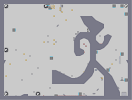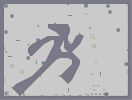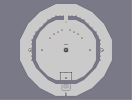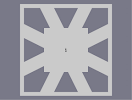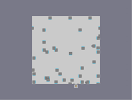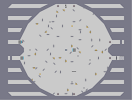the big mount-N the big mount-N #2 Down in One free tiles anyone? thwumpty dumpty 1 minute wonder

Pages: (5) [ 1 ] 2 3 4 5

### did you know...

that Mare and Raigan are also fans of the foos. they have a map named after them (alone + easy target)

:D

is my hero.

### N art that even looks good close up...

Wow... just wow.
DUDE I F\$%%IN LOVE THAT SONG GREAT ART 5

so owned

### No, sorry

The object count would be too low.

### "IF I GIVE OYU A PICTURE OF ME WILL YOU MAKE IT?!?!?!?!?"

IF I GIVE YOU A PICTURE OF MY DICK WILL YOU MAKE?

### This map is angry with John McCain!

Vote Democrat! :D

### lol

it sorta looks like the dude from "My Name Is Earl"
very nice though 5/5

### Cool,

I don't know who he is, but I know the group. 4.5

### ...

I knew it looked like the guy from the Foo Fighters, but I couldn't quite place it...

wow amazing!

### For all those

who can't be bothered to look down the list, it's David Grohl from the Foo Fighters (My Hero is one of their songs).

5/5

### NO

it's definately chuck norris

### ...

he looks like Tom Cruise to me..

I GOT IT! WOOO!

Sorry...

### I know

It's Che Guevara. I WIN :P

### nice

dave grohl rocks
Not bam. he does not have a beird.

### Guess:

Either Bam Margera or Chuck Norris
lol

### THIS IS A REALLY BAD GUESS

i'm guessing Chuck Norris well that is what i thought b4 i saw the other guesses

### Who is this?

I dont recognize it, but its very good- 4.5/5

5/5

### 400th Rating

5/5 and the foo fighters are awesome.

### DAVE GROHL!

OMG DAVE GROHL! he was waaaaaaaaay better as drummer of Nirvana i mean listen to him at the start of nirvana's 'stay away' best drumming ever *RIP Kurt Cobain* foo fighters suck though... still one of the best N Art I've seen... as soon as i saw the picture you made my day... (because of Nirvana) Im a artist in real life but i cant draw for S*** on a computer... so i envy you for that... but honestly... and answer this truthfully... DO YOU HAVE A LIFE lol. nah im sure you do. ur just an artist with to much time on your hands... thats it yeah. we can pretend...

### nice

hate foo fighters though -_-

didnt mean it

this gets a 5/5 but i would give it like 50 if i could

### Yeah

The reason that the hair and beard are placed with the snap on is because it reduced the number of mine that were used. Having such a broad area filled in with no snap used an ungodly amount of mines that was killing my computer, so the snap took the total number of mines down so that I could actually complete the map. ;)

### I don't like how the hair and beard are so red

It destroys the texturing. Other than that, it's great!

### well..

I had one very quiet day at uni. Yeah, Uni, I am doing something with my life actually. :P#